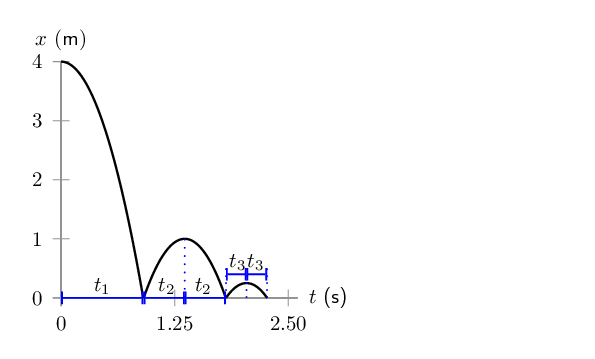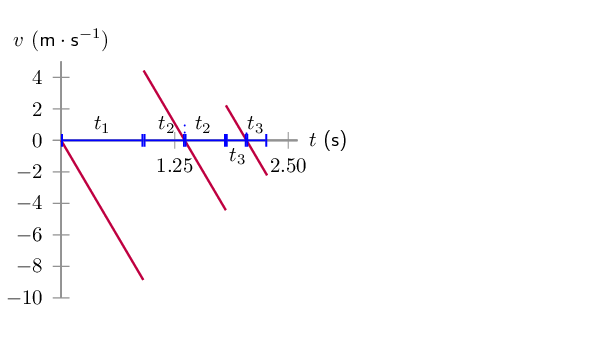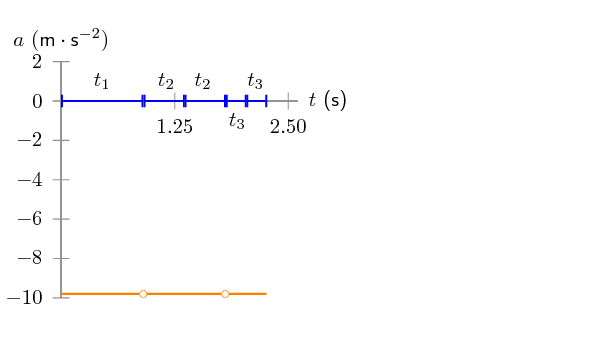Home Practice
For learners and parents For teachers and schools
Textbooks
Full catalogue
Pricing SupportLog in

We think you are located in United States. Is this correct?

# 3.2 Vertical projectile motion

## 3.2 Vertical projectile motion (ESCJW)

In Grade 11, we studied the motion of objects in free fall and saw that such an object has a constant gravitational acceleration of $$\vec{g}$$. We now study the motion of objects that are moving upwards or downwards while experiencing a force due to gravity. We call this projectile motion. We will only consider the case where objects move vertically upwards and/or downwards - meaning that there is no horizontal displacement of the object, only vertical displacement.

When we talk about a projectile we mean a body or particle that is project or launched and then moves under the influence of only gravity.

### Motion in a gravitational field (ESCJX)

We know from Newton's Law of Universal Gravitation that an object in the Earth's gravitational field experiences a force pulling it towards the centre of the Earth. If this is the only force acting on the object then the object will accelerate towards the centre of the Earth.

A person standing on the Earth's surface will interpret this acceleration as objects always falling downwards.

In Grade 11, we showed that the value of the acceleration due to gravity can be treated as a having a constant magnitude of $$g=\text{9,8}\text{ m·s^{-2}}$$.

In reality, if you go very far away from the Earth's surface, the magnitude of $$\vec{g}$$ would change, but, for everyday problems, we can safely treat it as constant. We also ignore any effects that air resistance (drag) might have.

For the rest of this chapter we will deal only with the case where the force due to gravity is the only force acting on the projectile that is falling. Any projectile can be described as falling, even if its motion is upwards initially.

The initial velocity, $$\vec{v}_i$$, that an object has and the acceleration that it experiences are two different quantities. It is very important to remember that the gravitational acceleration is always towards the centre of the Earth and constant, regardless of the direction or magnitude of the velocity.

Projectiles moving upwards or downwards in the Earth's gravitational field always accelerate downwards with a constant acceleration $$\vec{g}$$. Note: non-zero acceleration means that the velocity is changing.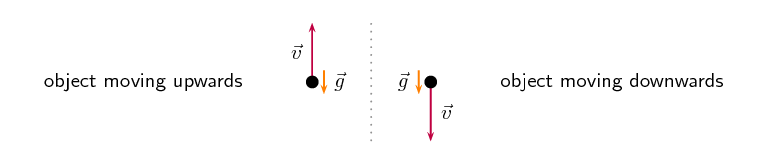Figure 3.1: Objects moving upwards or downwards in the Earth's gravitational field always accelerate towards the centre of the Earth. This looks like a downwards acceleration to someone standing on the Earth's surface. We don't draw both vectors on the object because we would be mixing velocity and acceleration which are two different physical quantities.

This means that if an object is moving upwards with some initial velocity in the vertical direction, the magnitude of the velocity in the vertical direction decreases until it stops ($$v=0$$  $$\text{m·s ^{-1}}$$) for an instant. The point at which the velocity is reduced to zero corresponds with the maximum height, $$h_{max}$$, that the object reaches. After this, the object starts to fall. It is very important to remember that the acceleration is constant but the velocity vector has changed in magnitude and direction. At the maximum height where the velocity is zero the acceleration is still $$\vec{g}$$.

Projectiles that have an initial velocity upwards will have zero velocity at their greatest height, $$h_{max}$$. The acceleration is still $$\vec{g}$$.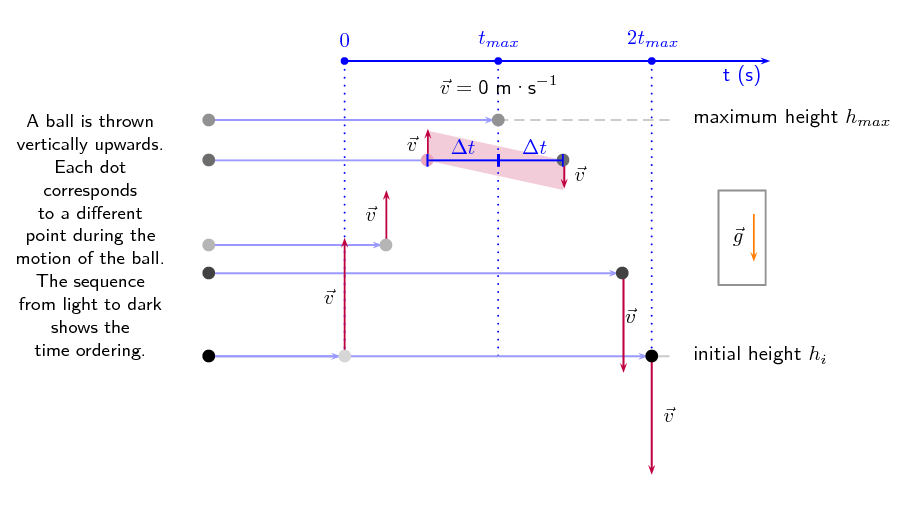Figure 3.2: (a) An object is thrown upwards from height $${h}_{i}$$. After time $${t}_{m}$$, the object reaches its maximum height, and starts to drop downwards. After a time $$2{t}_{m}$$ the object returns to height $${h}_{i}$$.

Consider an object thrown upwards from a vertical height $${h}_{i}$$. We have said that the object will travel upwards with decreasing vertical velocity until it stops, and then starts moving vertically downwards. The time that it takes for the object to fall back down to height $${h}_{i}$$ is the same as the time taken for the object to reach its maximum height from height $${h}_{i}$$. This is known as time symmetry. This is a consquence of the uniform acceleration the projectile experiences.

This has two implications for projectiles that pass through a point on both the upward and downward part of their motion when in free-fall:

• time symmetry: the time intervals during the upward motion and the downward motion are the same, for example it will take the same time to rise from initial position to maximum height as it will to drop back to the initial position. This applies to any point as shown by the pink dot in Figure 3.2. The time interval between the projectile passing the point and being at maximum height is the same, $$\Delta t$$.
• magnitude of velocity: the magnitude of the velocity at the same point on the upward and downward motion will be the same, the direction will be reversed, as indicated by the shaded region in Figure 3.2.

This is very useful when solving problems because if you have any information about the upward motion you can learn something about the downward motion as well and vice versa.

## Investigate the motion of a falling body

### Aim

To measure the position and time during motion and to use that data to plot a “Position vs Time” and “Velocity vs Time” graph from which we can calculate the magnitude for gravitational acceleration, $$\vec{g}$$.

### Apparatus

• ticker tape apparatus
• ticker timer
• tape
• graph paper
• ruler
• foam cushion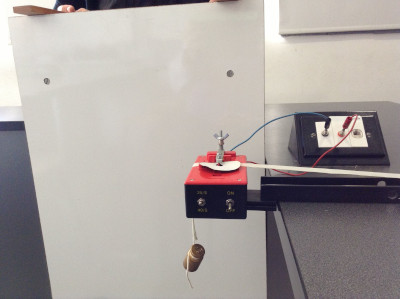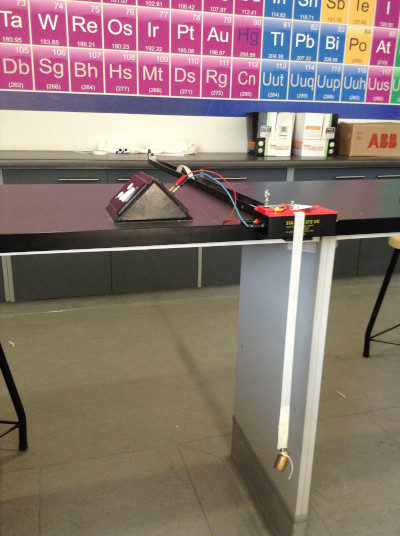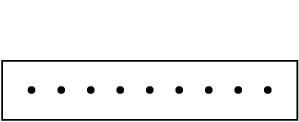Motion at constant velocity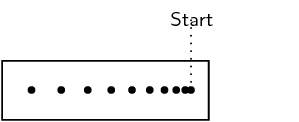Motion with increasing velocity

### Method

1. Work with a friend. Copy the table below into your workbook.

2. Clamp the timer to the table to allow the tape to fall through the staples on the timer such that the ticker tape will be perpendicular to the floor. Plug in the timer.
3. Thread a piece of ticker tape through the staples. Make sure the tape is under the carbon disc. The length of the tape should be the distance from the timer to the floor.
4. Using masking tape attach a 100 g mass to the end of the tape closest to the floor.
5. Position the foam cushion directly below the mass. (The cushion will protect your masses and your floor)
6. Hold the tape so that it is vertical and will easily pass through the staples.
7. Turn on the timer.
8. Let go of the tape with the mass, allowing it to drop to the floor.
9. Turn off the timer.
10. Repeat.
11. Take each tape and find the first clear dot nearest the mass end of the tape. Circle this first clear dot and label it as zero. Then count five dots and label the fifth dot as number 1. Count another 5 dots and label the fifth dot number 2. Continue this procedure.
12. On each piece of tape, measure the distance between successive dots. Note these distances in the table below.

13. Use the frequency of the ticker timer to work out the time intervals between successive dots. Note these times in the table below.

14. Work out the average of the two distance values between successive numbered dots.

15. The time interval between two dots is 0,02 s so the time between numbered dots is 0,1 s.
16. Use the average distance between numbered dots and the known time interval to calculate velocity.
17. Use the magnitude of the velocity and time values to plot a graph of “Velocity vs Time” onto graph paper. Stick the graph paper into your workbook.

18. Draw the best straight line through your data points.

19. Determine the slope of the straight line. This is the magnitude of the acceleration.

### Results

 Change in distance (m) Change in time (s) Magnitude of velocity ($$\text{m·s^{-1}}$$)

### Discussion

Describe the motion of the mass using the graphs.

### Equations of motion (ESCJY)

The equations of rectilinear motion that you learnt about in Grade 10 can be used for vertical projectile motion, with acceleration from gravity: $$\vec{a}=\vec{g}$$. Why, because the equations of rectilinear motion can be applied to any motion in a straight line with constant acceleration. If we are only considering vertical motion (up and down) it is motion in a straight line and the acceleration, $$\vec{g}$$, is constant and along the same line. We use the magnitude of $$g= \text{9,8}\text{ m·s ^{-2}}$$ for our calculations.

Now would be a good time to revise both rectilinear motion and quadratic equations from Grade 10.

Remember that when you use these equations, you are dealing with vectors which have magnitude and direction. Therefore, you need to decide which direction will be the positive direction so that your vectors have the correct signs. The equations of motion that you learnt about in Grade 10 are:

\begin{align*} \vec{v}_{f}& = \vec{v}_{i}+\vec{a}t \\ \Delta \vec{x}& = \frac{\left(\vec{v}_{i}+\vec{v}_{f}\right)}{2}t \\ \Delta \vec{x}& = \vec{v}_{i}t+\frac{1}{2}\vec{a}{t}^{2} \\ \vec{v}_{f}^{2}& = \vec{v}_{i}^{2}+2\vec{a}\Delta\vec{ x } \end{align*}

In the case of vertical projectile motion, we know that the only force we will consider is gravity and therefore the acceleration will be $$\vec{a}=\vec{g}$$.

\begin{align*} \vec{v}_{f}& = \vec{v}_{i}+\vec{g}t \\ \Delta \vec{x}& = \frac{\left(\vec{v}_{i}+\vec{v}_{f}\right)}{2}t \\ \Delta \vec{x}& = \vec{v}_{i}t+\frac{1}{2}\vec{g}{t}^{2} \\ \vec{v}_{f}^{2}& = \vec{v}_{i}^{2}+2\vec{g}\Delta x \end{align*}\begin{align*} &\text{where}\\ \vec{v}_{i}& = \text{initial velocity} \text{(}\text{m}·{\text{s}}^{-1}\text{)} \text{at start} \\ \vec{v}_{f}& = \text{final velocity} \text{(}\text{m}·{\text{s}}^{-1}\text{)} \text{at time} t \\ \Delta \vec{x}& = \text{change in vertical position} \text{(m)} \\ t& = \text{time} \text{(s)} \\ \Delta t& = \text{time interval} \text{(s)} \\ \vec{a}&=\vec{g} = \text{acceleration due to gravity} \text{(}\text{m}·{\text{s}}^{-2}\text{)} \end{align*}

$$\Delta \vec{x}$$ is displacement, the change in position. If the coordinate system you choose isn't centred at the point from which the motion starts (i.e. you don't have $$\vec{x}_i=0$$) then you must remember that the displacement and the final position are not the same. The position is given by $$\vec{x}_f=\vec{x}_i+\Delta \vec{x}$$. If $$\vec{x}_i=0$$ then $$\vec{x}_f=(0)+\Delta \vec{x}$$.

Now we can apply this mathematical description of vertical motion to solving problems.

## Worked example 1: Projectile motion

A ball is thrown vertically upwards with an initial velocity of $$\text{10}$$ $$\text{m·s ^{-1}}$$. A photograph (shown) is taken when the ball is $$\text{1,5}$$ $$\text{m}$$ above the point of release.1. Determine the maximum height above the thrower's hand reached by the ball.

2. Determine the time it takes the ball to reach its maximum height.

3. Determine the time(s) when the ball will be at the position shown in the photograph.

### Identify what is required and what is given

We are required to determine the maximum height reached by the ball and how long it takes to reach this height. We are given the initial velocity $$\vec{v}_{i}=\text{10}\text{ m·s^{-1}}$$ upwards and the acceleration due to gravity $$\vec{g}= \text{9,8}\text{ m·s ^{-2}}$$ downwards. We also need to determine when the ball is at the position shown in the photograph.

The ball is thrown straight up and will reach a maximum height, at which point its velocity will be zero, before beginning to fall downwards. The height of the ball in the photograph is given as $$\text{1,5}$$ $$\text{m}$$ above the initial point. Adding the information to the picture helps us understand what is going on more effectively: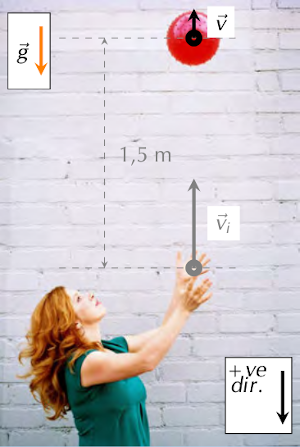### Determine how to approach the problem

Even though there are a number of questions to answer, the best approach is to work through them one at a time.

First, as with rectilinear motion in Grade 10, select a positive direction. We choose down as positive and remember to keep this sign convention for the whole problem. This will allow us to analyse the information given and determine directions and signs. We know that at the maximum height the velocity of the ball is $$\text{0}$$ $$\text{m·s ^{-1}}$$. We therefore have the following:

• $$\vec{v}_{i}= -\text{10}\text{ m·s ^{-1}}$$(it is negative because we chose downwards as positive)

• $$\vec{v}_{f}= \text{0}\text{ m·s ^{-1}}$$

• $$\vec{g}= \text{9,8}\text{ m·s ^{-2}}$$

We know that the position shown ($$\text{1,5}$$ $$\text{m}$$ above the point of release) will be passed as the ball rises to its maximum height and then again as the ball falls downwards.

### Identify the appropriate equation to determine the maximum height

We can use $$\vec{v}_{f}^{2}=\vec{v}_{i}^{2}+2\vec{g}\Delta \vec{x}$$ to solve for the height.

### Substitute the values into the equation and find the maximum height

\begin{align*} \vec{v}_{f}^{2}& = \vec{v}_{i}^{2}+2\vec{g}\Delta \vec{x} \\ {\left(0\right)}^{2}& = {\left( -\text{10}\right)}^{2}+\left(2\right)\left( \text{9,8}\right)\left(\Delta x\right) \\ -\text{100}& = \text{19,6}\Delta x \\ \Delta x& = -\text{5,102}\text{ m}\end{align*}

The value for the displacement will be negative because the displacement is upwards and we have chosen downward as positive (and upward as negative). The maximum height will be a positive number, $$h_m= \text{5,10}\text{ m}$$.

### Identify the appropriate equation to determine the time to reach maximum height

We know the values of $$\vec{v}_i$$, $$\vec{v}_f$$ and $$\vec{g}$$ so we can use: $$\vec{v}_{f}=\vec{v}_{i}+\vec{g}t$$ to solve for the time.

### Substitute the values in and find the time to reach maximum height

\begin{align*} \vec{v}_{f}& = \vec{v}_{i}+\vec{g}t \\ \text{0}& = -\text{10}+ \text{9,8}t \\ \text{10}& = \text{9,8}t \\ t& = \text{1,02}\text{ s}\end{align*}

### Determine the times at which the ball is in the position in the photograph

We know the displacement, initial velocity and the acceleration of the ball. We also expect to get two values as we know the ball will pass through the point twice.

We substitute our values into the equation $$\Delta \vec{x} = \vec{v}_{i}t+\frac{1}{2}\vec{g}{t}^{2}$$, which is a quadratic equation in time, so we expect to find two different solutions if we solve for time.

It is important to notice that we can use the value $$-\text{1,5}$$ $$\text{m}$$ as the displacement above the initial position.

\begin{align*} \Delta \vec{x} & = {v}_{i}t+\frac{1}{2}\vec{g}{t}^{2} \\ %& \text{We will solve for time using the quadratic formula} \\ \frac{1}{2}\vec{g}{t}^{2} + \vec{v}_{i}t - \Delta \vec{x} & = \text{0}\\ \frac{1}{2}( \text{9,8})t^2 + ( -\text{10})t - ( -\text{1,5}) & = \text{0}\\ \underbrace{( \text{4,9})}_{a}\underbrace{t^2}_{x^2} + \underbrace{( -\text{10})}_{b}\underbrace{t}_{x}+\underbrace{(\text{1,5})}_{c} & = 0 \\ t &= \frac{-b\pm\sqrt{b^2- \text{4}ac}}{ \text{2}a} \\ t &= \frac{-( -\text{10})\pm\sqrt{( -\text{10})^2- \text{4}( \text{4,9})( \text{1,5})}}{ \text{2}( \text{4,9})} \\ t &= \frac{ \text{10}\pm\sqrt{ \text{70,6}}}{ \text{9,8}} \\ t &= \frac{ \text{10}\pm \text{8,40238}}{ \text{9,8}} \\ t &= \text{1,02041}\pm \text{0,857386}\\ t = \text{0,16}\text{ s}\ & \text{or}\ t = \text{1,88}\text{ s}\end{align*}

The ball:

1. reaches a maximum height of $$\text{5,10}$$ $$\text{m}$$;
2. takes $$\text{1,02}$$ $$\text{s}$$ to reach the top; and
3. passes through the point in the photograph at $$t$$= $$\text{0,16}$$ $$\text{s}$$ on the way up and t = $$\text{1,88}$$ $$\text{s}$$ on the way down.

The use of a sign convention should not affect your result. As an exercise, repeat the previous worked example using the opposite sign convention to verify that you do get the same result.

## Worked example 2: Height of a projectile

A cricketer hits a cricket ball so that it goes vertically upwards. If the ball takes $$\text{10}$$ $$\text{s}$$ to return to the initial height, determine its maximum height above the initial position.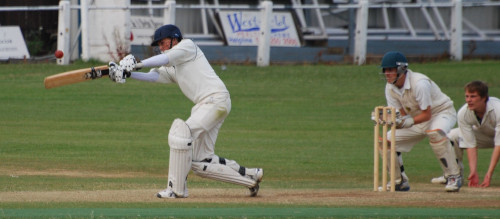### Identify what is required and what is given

We need to find how high the ball goes. We know that it takes $$\text{10}$$ $$\text{s}$$ to go up and down. We do not know what the initial velocity ($$\vec{v}_{i}$$) of the ball is.

After the batsman strikes the ball it goes directly upwards and the only force that will be acting on the ball will be the gravitational force. This information is represented in the photograph below: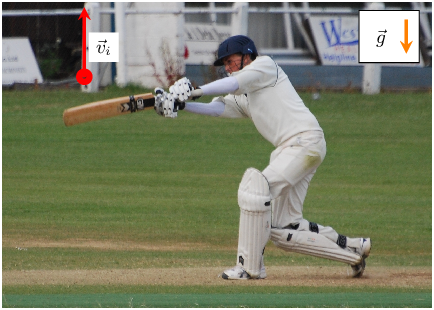### Determine how to approach the problem

For a problem like this it is useful to divide the motion into two parts. The first part of the motion consists of the upward motion of the ball with an initial velocity ($$\vec{v}_{i}$$) and a final velocity ($$\vec{v}_{f}=0$$) at the maximum height. The second part of the motion consists of the downward motion of the ball with an initial velocity ($$\vec{v}_{i}=0$$) and final velocity ($$\vec{v}_{f}$$) which is not yet known.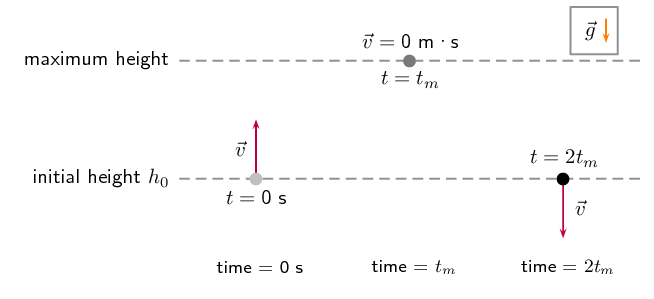Choose down as positive. We know that at the maximum height, the velocity of the ball is $$\text{0}$$ $$\text{m·s ^{-1}}$$. We also know that the ball takes the same time to reach its maximum height as it takes to travel from its maximum height to the initial position, due to time symmetry. The time taken is half the total time. Therefore, we have the following information for the second (downward) part of the motion of the ball:

• $$t=\text{5}\text{ s}$$ (half of the total time)

• $$\vec{v}_{\mathrm{top}}=\text{0}\text{ m·s^{-1}}$$

• $$\vec{g}= \text{9,8}\text{ m·s ^{-2}}$$ downwards

• $$\Delta \vec{x}=~?$$

### Find an appropriate equation to use

We do not know the final velocity of the ball coming down. We need to choose an equation that does not have $$\vec{v}_{f}$$ in it. We can use the following equation to solve for $$\Delta \vec{x}$$:

$\Delta \vec{x}=\vec{v}_{i}t+\frac{1}{2}\vec{g}{t}^{2}$

### Substitute values and find the height

\begin{align*} \Delta \vec{x}& = \left( \text{0}\right)\left( \text{5}\right)+\frac{ \text{1}}{ \text{2}}\left( \text{9,8}\right){\left( \text{5}\right)}^{2} \\ \Delta \vec{x}& = \text{122,5}\text{ m}~\text{downwards}\end{align*}

In the second motion, the displacement of the ball is $$\text{122,5}$$ $$\text{m}$$ downwards. This means that the height was $$h = \text{122,5}\text{ m}$$.

The ball reaches a maximum height of $$\text{122,5}$$ $$\text{m}$$.

## Worked example 3: Equal displacements [attribution: Sunil Kumar Singh]

A sequence of balls are successively dropped from the top of a tower. The time interval between the release of consecutive balls is equal. At the precise instant that the ninth ball (ball 9) is released, the first ball hits the ground. Which of the balls in the sequence is at $$\frac{3}{4}$$ of the height, $$h$$, of the tower when the first ball hits the ground?

### Understand what is happening

A ball is dropped from a tower. After a time, $$t_i$$, a second ball is dropped. After another time interval, $$t_i$$, a third ball is dropped. This means that when ball 3 is dropped the total time that has passed is $$2t_i$$.

After a third time interval, $$t_i$$, a fourth ball is dropped. This means that at the instant that ball 4 is dropped the total time that has passed is $$3t_i$$. This process continues until the ninth ball is dropped. At the same instant that the ninth ball is dropped, the first ball that was dropped strikes the ground. The ninth ball would have been released after 8 time intervals, therefore it took the first ball a time interval of $$8t_i$$ to hit the ground.

### Describe the events mathematically

As this is a vertical motion problem where everything is falling towards the ground, it is simplest to choose downwards as the positive direction.

The first ball hits the ground when the ninth ball is dropped. This means that the first ball has fallen for a total time of $$( \text{9}- \text{1})t_i = \text{8}t_i$$. The displacement of the first ball is also described by the equation $$\Delta \vec{x}=\vec{v}_{i}t+\frac{1}{2}\vec{g}t^2$$. The balls are dropped so their initial velocity is zero, $$\vec{v}_{i}=0$$ and the we know the time for the first ball to fall the height of the tower therefore, in the case of the first ball, we have:

\begin{align*} \Delta \vec{x} &=\vec{v}_{i}t+\frac{1}{2}\vec{g}t^2 \\ h &=\frac{1}{2}(\text{9,8})(\text{8}t_i)^2 \end{align*}

Let the $$n$$th ball be the ball that is at $$\frac{3}{4}$$ of the height, $$h$$, of the tower when the first ball strikes the ground. The $$n$$th ball will have fall for a time of $$(n-1)t_i$$. If it is $$\frac{3}{4}$$ of the way up the tower it will have fall a distance of $$\frac{1}{4}h$$. This motion is also described by $$\Delta \vec{x}=\vec{v}_{i}t+\frac{1}{2}\vec{g}t^2$$, and therefore we know, in the case of the $$n$$th ball, we have:

\begin{align*} \Delta \vec{x} &=\vec{v}_{i}t+\frac{1}{2}\vec{g}t^2 \\ \frac{1}{4}h &=\frac{1}{2}(\text{9,8})((n-\text{1})t_i)^2 \\ h &=2(\text{9,8})((n-\text{1})t_i)^2 \end{align*}

We can't solve for $$n$$ from this final equation because it will contain $$h$$ and $$t_i$$. We can use the two equations we have to eliminate $$h$$. Both equations are written in the form $$h=...$$ therefore we can equate them eliminating $$h$$:

\begin{align*} \frac{1}{2}(\text{9,8})(\text{8}t_i)^2 & = 2(\text{9,8})((n-\text{1})t_i)^2 \\ \frac{1}{2}(\text{9,8})\text{8}^2t_i^2 & = 2(\text{9,8})(n-\text{1})^2t_i^2 \\ \left(\frac{2}{(\text{9,8})t_i^2}\right)\times\frac{1}{2}(\text{9,8})\text{8}^2t_i^2 & = \left(\frac{2}{(\text{9,8})t_i^2}\right)\times 2(\text{9,8})(n-\text{1})^2t_i^2 \\ \text{8}^2 & = 4(n-\text{1})^2 \\ \text{8} & = 2(n-\text{1}) \\ \text{4} & = (n-\text{1}) \\ n & = 5 \end{align*}

We know that $$n$$ must be a positive integer which is why we use the positive roots when we take the square root above.

The fifth ball is at $$\frac{3}{4}$$ of the height of the tower when the first ball strikes the ground.

## Equations of motion

Textbook Exercise 3.1

A cricketer hits a cricket ball straight up into the air. The cricket ball has an initial velocity of $$\text{20}$$ $$\text{m·s ^{-1}}$$ vertically upwards.

1. What height does the ball reach before it stops to fall back to the ground.

2. How long has the ball been in the air for when it reaches that maximum height?

1. Note that the final velocity is 0 at the maximum height that the ball reaches. (Take upwards as +ve direction.)

\begin{align*} \vec{v}_{f}^{2} &=\vec{v}_i^{2} + 2\vec{g} \Delta\vec{x}\\ \Delta\vec{x}&=\frac{\vec{v}_i^{2}}{2\vec{g}} \\ &=\frac{-(\text{20}^{2})}{(\text{2})(-\text{9,8})} \\ &= \text{20,41}\text{ m} \end{align*}
2. \begin{align*} \vec{v}_{f}& = \vec{v}_{i} +\vec{g}t \\ t &= \frac{\vec{v}_{f}- \vec{v}_{i}}{\vec{g}} \\ &= \frac{\text{0}-\text{20}}{-\text{9,8}}\\ &=\text{2,04}\text{ s} \end{align*}
1. $$\text{20,41}$$ $$\text{m}$$
2. $$\text{2,04}$$ $$\text{s}$$

Zingi throws a tennis ball straight up into the air. It reaches a height of $$\text{80}$$ $$\text{cm}$$.

1. Determine the initial velocity of the tennis ball.

2. How long does the ball take to reach its maximum height?

1. We first convert the height given to m:

$$\frac{\text{80}}{\text{100}}=\text{0,8}\text{ m}$$ \begin{align*} \vec{v}_{f}^2 &= \vec{v}_{i}^2 + 2\vec{g}\Delta{\vec{x}} \\ 0 &= \vec{v}_{i}^2 + 2(-\text{9,8})(\text{0,8}) \\ \vec{v}_{i}^2 &= \text{15,68} \\ \vec{v}_{i} &= \text{3,96}\text{ m·s$^{-1}$} \end{align*}
2. \begin{align*} \vec{v}_{f} &= \vec{v}_{i} + \vec{g}t \\ \text{0}&= \text{3,96} + (-\text{9,8})(t) \\ \text{9,8}t &=\text{3,96} \\ t &= \text{0,4}\text{ s} \end{align*}
1. $$\text{3,96}$$ $$\text{m·s^{-1}}$$ upwards
2. $$\text{0,4}$$ $$\text{s}$$

A tourist takes a trip in a hot air balloon. The hot air balloon is ascending (moving up) at a velocity of $$\text{4}$$ $$\text{m·s ^{-1}}$$ vertically upwards. He accidentally drops his camera over the side of the balloon's basket, at a height of $$\text{20}$$ $$\text{m}$$. Calculate the velocity with which the camera hits the ground.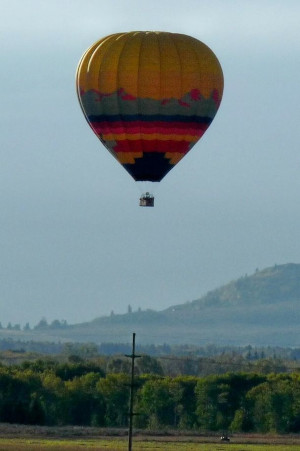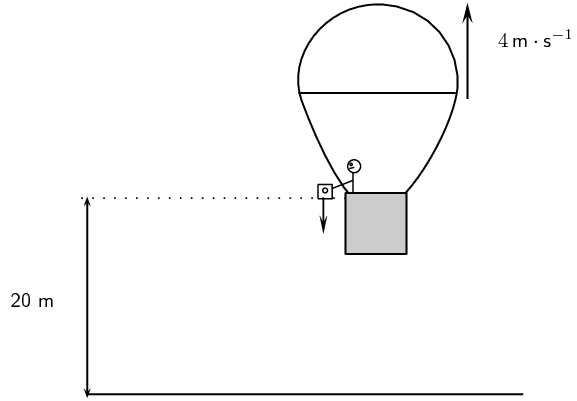Draw a rough sketch:

We now calculate the additional height above the ground that the camera reaches:

\begin{align*} \vec{v}_{f}^2 &= \vec{v}_{i}^2 + 2\vec{g}\Delta{\vec{x}} \\ \Delta{\vec{x}} &= \frac{\text{14}^{2}}{\text{2}(\text{9,8})} \\ &= \text{0,82}\text{ m} \end{align*}

So the total height above the ground is $$\text{20,82}$$ $$\text{m}$$.

\begin{align*} \vec{v}_{f}^2 &= \vec{v}_{i}^2 + 2\vec{g}\Delta{\vec{x}} \\ \vec{v}_{f}^2 &= \text{2}(\text{9,8})(\text{20,82}) = \text{408,072} \\ \vec{v}_{f} &= \text{20,2}\text{ m·s$^{-1}$} \end{align*} $$\text{20,2}$$ $$\text{m·s^{-1}}$$

A ball is dropped vertically from a tower. If the vertical distance covered in the last second is equal to the distance covered in first three seconds, find the height of the tower.

Let us consider that the ball covers the height $$y_n$$ in $$n^{\text{th}}$$ second. Then, the distance covered in the $$n^{\text{th}}$$ second is given as :

\begin{align*} y_n & = u + \frac{g}{2} \times (2n - 1) \\ & = 0 + \frac{\text{9,8}}{2} \times (2n - 1) \\ & = \text{9,8}n - 5 \end{align*}

On the other hand, the distance covered in first 3 seconds is :

\begin{align*} y_3 & = ut + \frac{1}{2}gt^{2} \\ & = 0 + \frac{1}{2} \times \text{9,8} \times 3^{2} \\ & = \text{44,1}\text{ m} \end{align*}

According to question,

\begin{align*} y_n & = y_3 \\ \text{9,8}n - 5 & = 44,1 \\ n & = \text{5,01}\text{ s} \end{align*}

Therefore, the height of the tower is :

\begin{align*} y & = \frac{1}{2} \times \text{9,8} \times \text{5,01}^2 \\ & = \text{123}\text{ m} \end{align*}

### Graphs of vertical projectile motion (ESCJZ)

We can describe vertical projectile motion through a series of graphs: position, velocity and acceleration versus time graphs. This isn't new, in Grade 10 you learnt about the graphs for describing rectilinear motion with constant acceleration. In the previous section we motivated for using the same equations of motion as in rectilinear motion. Therefore, everything you learnt about the graphs describing rectilinear motion is applicable when describing vertical projectile motion.

Now would be a good time to revise the section on graphs in rectilinear motion from Grade 10.

Remember that: $\vec{a}=\frac{\Delta \vec{v}}{\Delta t}$ $\vec{v}=\frac{\Delta \vec{x}}{\Delta t}$ For the graphs of these quantities the slope and the area under a graph can tell us about changes in other quantities. As a reminder, here is a summary table, from Grade 10 rectilinear motion, about which information we can derive from the slope and the area of various graphs: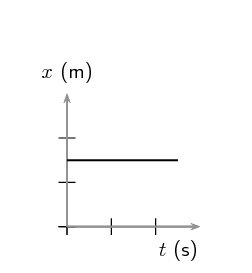Figure 3.3: Position vs time, velocity vs time and acceleration vs time graphs.

The graphs are the graphical representations of the equations of motion. This means that if you have the graph for one of position, velocity or acceleration you should be able to write down the corresponding equation and vice versa.

The type of graphs is related to the equation of motion: position is a parabola, velocity is a straight line and acceleration is a constant. The characteristic features then depend on the sign convention and the specific values of the problem.

To illustrate the variation, we consider three separate cases: (1) the object has an initial velocity in the upward direction (opposite direction to $$\vec{g}$$), (2) the object falls from rest (no initial velocity), and (3) the object has an initial velocity in the downward direction (same direction as $$\vec{g}$$).

Important: the horizontal axis in these graphs is time, not space.

#### Case 1: Initial velocity upwards

Consider an object undergoing the following motion:

1. at time $$t=\text{0}\text{ s}$$ the object has an initial position, $$\vec{x}_i=0$$, and an initial upward velocity of magnitude $$v_i$$,
2. the object reaches a maximum height, $$h_m$$, above the ground,
3. the object then falls down to a final position, $$\vec{x}_f$$, with a final velocity $$v_f$$ downward at time = $$t_f$$.

The equation for position is given by: $$\vec{x} = \vec{x}_i + \vec{v}_{i}t+\frac{1}{2}\vec{g}{t}^{2}$$. We have to choose a positive direction as before. We choose upwards as positive. The position versus time graph for the motion described is shown below:

It is important to notice that we are plotting position versus time and the initial position, $$\vec{x}_{i}$$, is not necessarily zero. The position as a function of time will be given by $$\vec{x}_f = \vec{x}_{i} + \vec{v}_{i}t+\frac{1}{2}\vec{g}{t}^{2}$$. The initial position, $$\vec{x}_{i}$$, will shift the graph of $$\vec{x}$$ up or down by a constant value. Whether it is an upward or downward shift will depend on which direction was chosen as the positive direction. The magnitude of the shift will depend on the choice of origin for the coordinate system (frame of reference).

The second graph is a linear function showing the velocity as a function of time: $$\vec{v}_{f} = \vec{v}_{i}+\vec{g}t$$. Note that we chose upwards as our positive direction therefore the acceleration due to gravity will be negative. The slope (i.e. the coefficient of $$t$$) of the velocity versus time graph is the acceleration and the slope is negative which is consistent with our expectations after choosing upwards as the positive direction.

The final plot is a graph of acceleration versus time, and is constant because the magnitude and direction of the acceleration due to gravity are constant.

#### Cases 2 and 3: Initial velocity zero or downwards

We start by choosing a direction as the positive direction. We want to compare the graphs with the first case, so we will choose the same direction (upwards) to be the positive direction.

The one thing that remains the same is the acceleration, $$\vec{g}$$, due to gravity. Therefore:

If an object starts from rest (for example is dropped), then the initial velocity is zero. We know that there is constant acceleration and hence the graph of velocity versus time looks like this: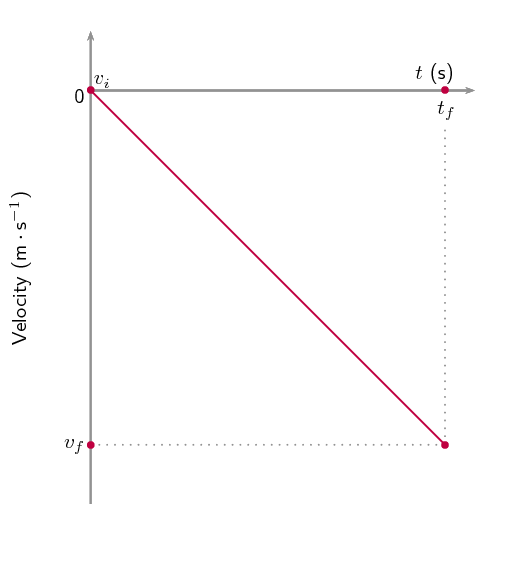If the object starts with an initial velocity downwards the velocity graph looks like: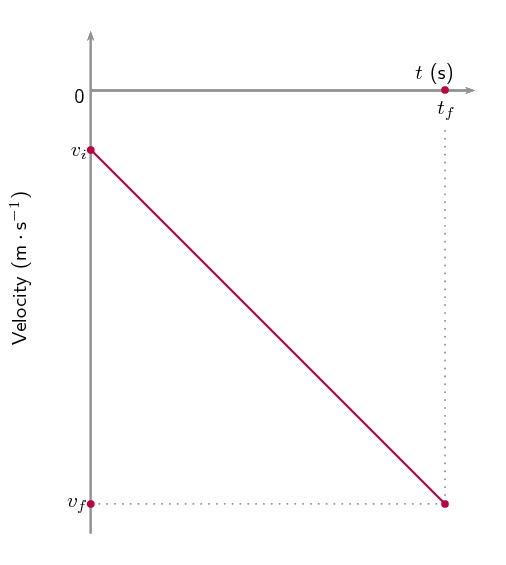It is important to notice that the slope of all the velocity versus time graphs has the same magnitude. If the same direction is chosen as positive then the slope has the same magnitude AND the same sign (direction). The difference in these graphs is related to the initial velocity, $$\vec{v}_{i}$$. The magnitude of the slope of the graph is the magnitude of the acceleration.

For the case where the object falls from rest, the equation for position is $$\vec{x} = \vec{x}_i +\underbrace{\vec{v}_{i}t}_{\text{0}}+\frac{1}{2}\vec{g}{t}^{2}$$ and the graph of the position versus time looks like this: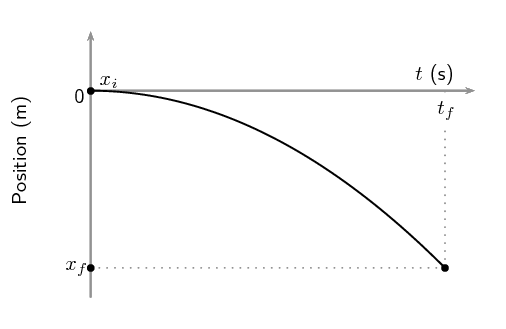For motion with an initial velocity in the negative direction, the graph of displacement versus time will be a narrower parabola as compared to the case where the object falls from rest. This is shown in this graph where the initial velocity is in the negative direction: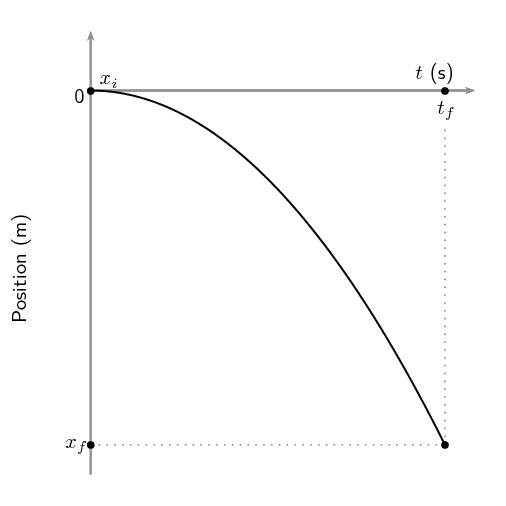The primary difference in features between the cases we've looked at is that for Case 1, the position graph has a maximum positive displacement (it has a peak), while in Cases 2 and 3, where the initial velocity is negative, the displacement is always negative.

You may be wondering if the final position is always the same as the initial position, as we had for our Case 1 example. That is not necessarily true. If an object is thrown up from the edge of a cliff, the initial position of the object will be the height of the cliff but the final position will be at the base of the cliff. If we choose the point from which the object is thrown as the origin of our coordinate system then the graph will look like this: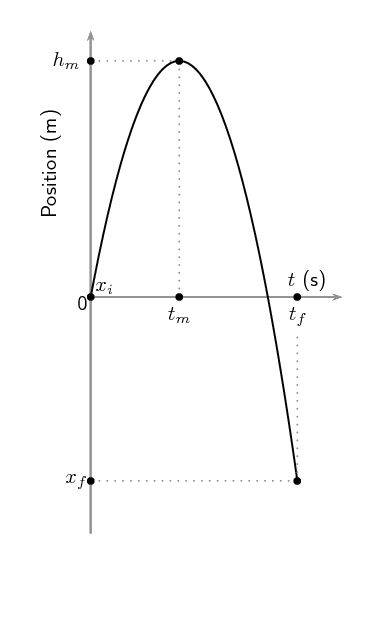If we choose to centre our coordinate system at the base of the cliff then the graph will look like this, because the initial displacement is the height of the cliff: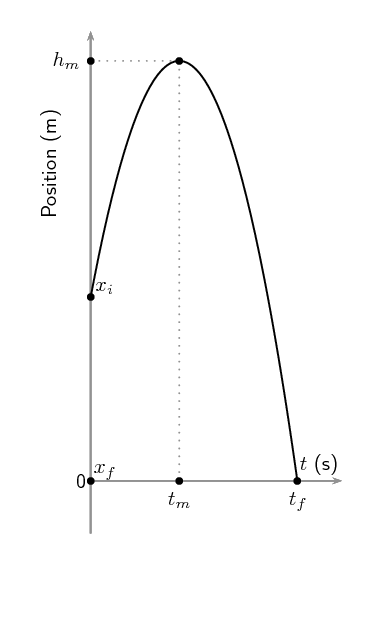## Worked example 4: Drawing graphs of projectile motion

Stanley is standing on a balcony $$\text{20}$$ $$\text{m}$$ above the ground. Stanley tosses a rubber ball upwards with an initial velocity of $$\text{4,9}$$ $$\text{m·s ^{-1}}$$. The ball travels upwards to a maximum height, and then falls to the ground. Draw graphs of position vs time, velocity vs time and acceleration vs time. Choose upwards as the positive direction.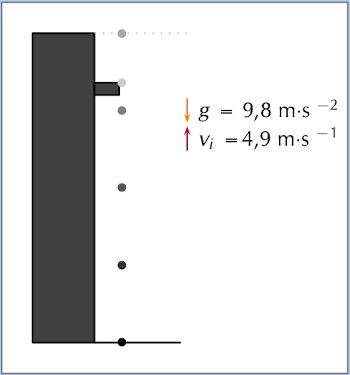### Determine what is required

We are required to draw graphs of:

1. $$\vec{x}$$ vs $$t$$

2. $$\vec{v}$$ vs $$t$$

3. $$\vec{a}$$ vs $$t$$

### Determine how to approach the problem

There are two parts to the motion of the ball:

1. ball travelling upwards from the building

2. ball falling to the ground

We examine each of these parts separately. To be able to draw the graphs, we need to determine the time taken and displacement for each of the motions.

### Find the height and the time taken for the first motion

For the first part of the motion we have:

• $$\vec{v}_{i}= \text{+4,9}\text{ m·s ^{-1}}$$

• $$\vec{v}_{f}= \text{0}\text{ m·s ^{-1}}$$

• $$\vec{g}= -\text{9,8}\text{ m·s ^{-2}}$$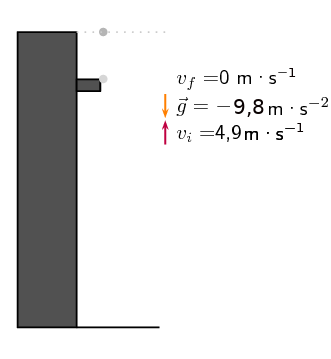Therefore we can use $$\vec{v}_{f}^{2}=\vec{v}_{i}^{2}+2\vec{g}\Delta \vec{x}$$ to solve for the height and $$\vec{v}_{f}=\vec{v}_{i}+\vec{g}t$$ to solve for the time.

\begin{align*} \vec{v}_{f}^{2}& = \vec{v}_{i}^{2}+2\vec{g}\Delta \vec{x} \\ {\left(0\right)}^{2}& = {\left( \text{4,9}\right)}^{2}+2\times \left(-\text{9,8}\right)\times \Delta \vec{x} \\ \text{19,6}\Delta x& = {\left(\text{4,9}\right)}^{2} \\ \Delta \vec{x}& = + \text{1,225}\text{ m} \\ h & = \Delta x = \text{1,225}\text{ m} \end{align*}\begin{align*} \vec{v}_{f}& = \vec{v}_{i}+\vec{g}t \\ 0& = \text{4,9}+\left( -\text{9,8}\right)\times t \\ \text{9,8}t& = \text{4,9}\\ t& = \text{0,5}\text{ s} \end{align*}

### Find the height and the time taken for the second motion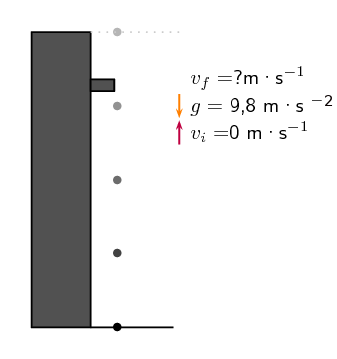For the second part of the motion we have:

• $$\vec{v}_{i}=\text{0}\text{ m·s^{-1}}$$

• $$\Delta \vec{x}=-\left(\text{20}+\text{1,225}\right) \text{m}$$

• $$\vec{g}= -\text{9,8}\text{ m·s ^{-2}}$$

Therefore we can use $$\Delta \vec{x}=\vec{v}_{i}t+\frac{1}{2}\vec{g}{t}^{2}$$ to solve for the time.

\begin{align*} \Delta \vec{x}& = \vec{v}_{i}t+\frac{1}{2}\vec{g}{t}^{2} \\ -\left(\text{20}+\text{1,225}\right)& = \left(0\right)\times t+\frac{1}{2}\times \left( -\text{9,8}\right)\times {t}^{2} \\ -\text{21,225}& = 0 -\text{4,9}{t}^{2} \\ {t}^{2}& = \text{4,33163}... \\ t& = \text{2,08125}\text{ s} \end{align*}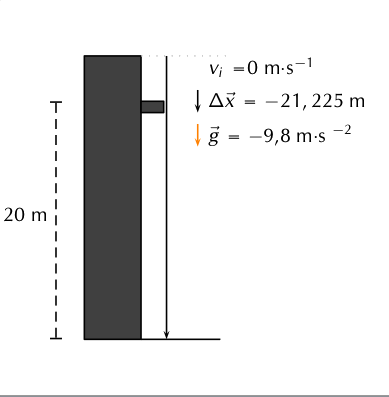### Graph of position vs time

The ball starts from a position of $$\text{20}$$ $$\text{m}$$ (at t = $$\text{0}$$ $$\text{s}$$) from the ground and moves upwards until it reaches $$\text{20}$$+$$\text{1,225}$$ $$\text{m}$$ (at $$t=\text{0,5}\text{ s}$$). It then falls back to $$\text{20}$$ $$\text{m}$$ (at $$t=\text{0,5}+\text{0,5}= \text{1,0}\text{s}$$) and then falls to the ground, $$\Delta \vec{x}=\text{0}\text{ m}$$ (at $$t=\text{0,5}+\text{2,08}=\text{2,58}~\text{s}$$).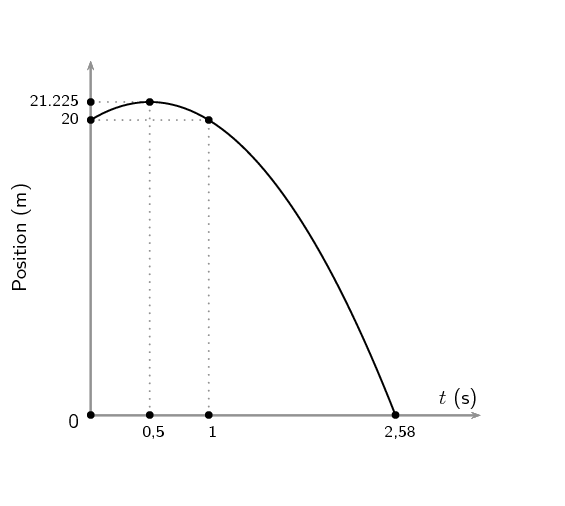### Graph of velocity vs time

The ball starts off with a velocity of $$\text{+4,9}$$ $$\text{m·s ^{-1}}$$ at t = $$\text{0}$$ $$\text{s}$$, it then reaches a velocity of $$\text{0}$$ $$\text{m·s ^{-1}}$$ at t = $$\text{0,5}$$ $$\text{s}$$. It stops and falls back to the Earth. At t = $$\text{1,0}$$ $$\text{s}$$ (i.e. after a further $$\text{0,5}$$ $$\text{s}$$) it has a velocity of $$-\text{4,9}$$ $$\text{m·s ^{-1}}$$. This is the same as the initial upwards velocity but it is downwards. It carries on at constant acceleration until $$t=\text{2,58}\text{ s}$$. In other words, the velocity graph will be a straight line. The final velocity of the ball can be calculated as follows:

\begin{align*} \vec{v}_{f}& = \vec{v}_{i}+\vec{g}t \\ & = 0+\left( -\text{9,8}\right)\left( \text{2,08}...\right) \\ & = -\text{20,396}... \text{m}·{\text{s}}^{-1} \end{align*}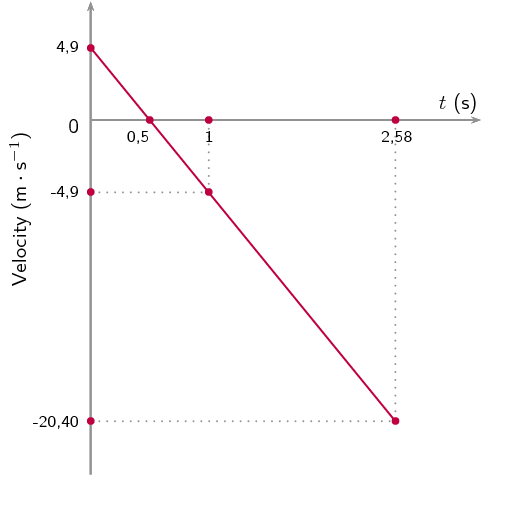### Graph of $$a$$ vs $$t$$

We chose upwards to be positive. The acceleration of the ball is downward, $$\vec{g}= \text{9,8}\text{ m·s ^{-2}}$$ downwards. Because the acceleration is constant throughout the motion, the graph looks like this: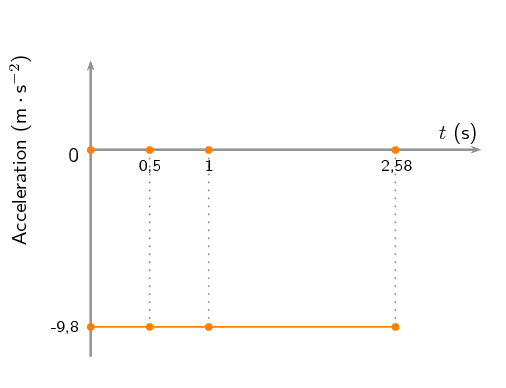## Worked example 5: Analysing graphs of projectile motion

The graph below (not drawn to scale) shows the motion of a tennis ball that was thrown vertically upwards from an open window some distance from the ground. It takes the ball $$\text{0,2}$$ $$\text{s}$$to reach its highest point before falling back to the ground. Study the graph given and calculate:

1. how high the window is above the ground.

2. the time it takes the ball to reach the maximum height.

3. the initial velocity of the ball.

4. the maximum height that the ball reaches.

5. the final velocity of the ball when it reaches the ground.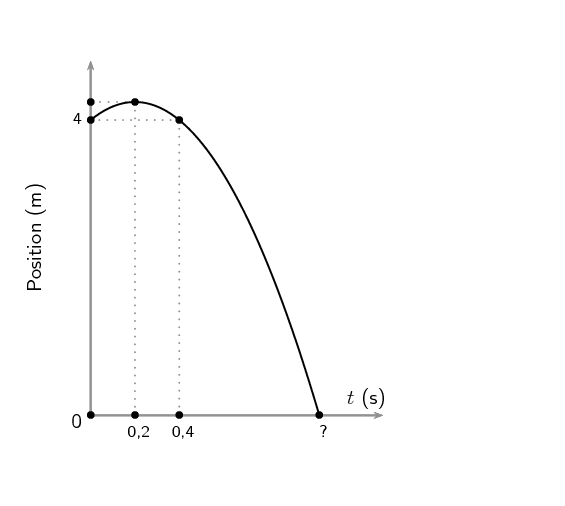### Find the height of the window

The initial position of the ball will tell us how high the window is. From the y-axis on the graph we can see that the ball is $$\text{4}$$ $$\text{m}$$ from the ground.

The window is therefore $$\text{4}$$ $$\text{m}$$ above the ground.

### Find the time taken to reach the maximum height

The maximum height is where the position vs time graph reaches its maximum position. This is when t = $$\text{0,2}$$ $$\text{s}$$.

It takes the ball $$\text{0,2}$$ $$\text{s}$$ to reach the maximum height.

### Find the initial velocity ( $$\vec{v}_{i}$$) of the ball

To find the initial velocity we only look at the first part of the motion of the ball. That is from when the ball is released until it reaches its maximum height. We have the following for this: In this case, let's choose upwards as positive.

\begin{align*} t& = \text{0,2} \text{s} \\ \vec{g}& = \text{9,8}\text{ m·s $^{-2}$}\\ \vec{v}_{f}& = 0 \text{m}·{\text{s}}^{-1} \text{(because the ball stops)} \end{align*}

To calculate the initial velocity of the ball ( $$\vec{v}_{i}$$), we use:

\begin{align*} \vec{v}_{f}& = \vec{v}_{i}+\vec{g}t \\ 0& = \vec{v}_{i}+\left( -\text{9,8}\right)\left( \text{0,2}\right) \\ {v}_{i}& = \text{1,96} \text{m}·{\text{s}}^{-1} \end{align*}

The initial velocity of the ball is $$\text{1,96}$$ $$\text{m·s ^{-1}}$$upwards.

### Find the maximum height of the ball

To find the maximum height we look at the initial motion of the ball. We have the following:

\begin{align*} t& = \text{0,2} \text{s} \\ \vec{g}& = \text{9,8}\text{ m·s $^{-2}$}\\ \vec{v}_{f}& = \text{0} \text{m}·{\text{s}}^{-1}~\text{(because the ball stops)} \\ \vec{v}_{i}& = \text{+1,96} \text{m}·{\text{s}}^{-1}\text{(calculated above)} \end{align*}

To calculate the displacement from the window to the maximum height ($$\Delta x$$) we use:

\begin{align*} \Delta \vec{x}& = \vec{v}_{i}t+\frac{1}{2}\vec{g}{t}^{2} \\ \Delta \vec{x}& = \left( \text{1,96}\right)\left( \text{0,2}\right)+\frac{1}{2}\left( -\text{9,8}\right){\left( \text{0,2}\right)}^{2} \\ \Delta \vec{x}& = \text{0,196} \text{m} \end{align*}

The maximum height of the ball is $$\left(\text{4}+\text{0,196}\right)= \text{4,196} \text{m}$$ above the ground.

### Find the final velocity ( $$\vec{v}_{f}$$) of the ball

To find the final velocity of the ball we look at the second part of the motion. For this we have:

\begin{align*} \Delta \vec{x}& = -\text{4,196} \text{m}~\text{(because upwards is positive)} \\ \vec{g}& = -\text{9,8}\text{ m·s $^{-2}$}\\ \vec{v}_{i}& = \text{0} \text{m}·{\text{s}}^{-1} \end{align*}

We can use $$\vec{v}^2_{f}=\vec{v}^2_{i}+2\vec{g}\Delta\vec{x}$$ to calculate the final velocity of the ball.

\begin{align*} \vec{v}^2_{f}=\vec{v}^2_{i}+2\vec{g}\Delta\vec{x} \\ \vec{v}^2_{f}& = {\left(0\right)}^{2}+2\left( -\text{9,8}\right)\left( -\text{4,196}\right) \\ \vec{v}^2_{f}& = \text{82,2416}\\ \vec{v}_{f}& = \text{9,0687}\text{ m·s$^{-1}$}~\text{downwards} \end{align*}

The final velocity of the ball is $$\text{9,07}$$ $$\text{m·s ^{-1}}$$ downwards.

## Worked example 6: Describing projectile motion

A cricketer hits a cricket ball from the ground and the following graph of velocity vs time was drawn. Upwards was taken as positive. Study the graph and follow the instructions below:

1. Describe the motion of the ball according to the graph.

2. Draw a sketch graph of the corresponding position-time graph. Label the axes.

3. Draw a sketch graph of the corresponding acceleration-time graph. Label the axes.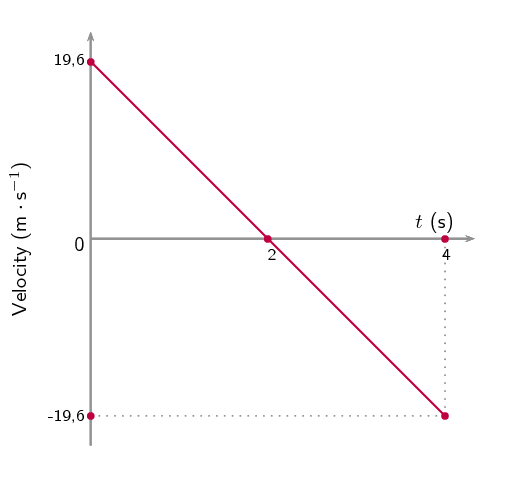### Describe the motion of the ball

We need to study the velocity-time graph to answer this question. We will break the motion of the ball up into two time zones: $$t=\text{0}\text{ s}$$ to $$t=\text{2}\text{ s}$$ and $$t=\text{2}\text{ s}$$ to $$t=\text{4}\text{ s}$$.

From $$t=\text{0}\text{ s}$$ to $$t=\text{2}\text{ s}$$ the following happens:

The ball starts to move at an initial velocity of $$\text{19,6}$$ $$\text{m·s ^{-1}}$$ and decreases its velocity to $$\text{0}$$ $$\text{m·s ^{-1}}$$ at $$t=\text{2}\text{ s}$$. At $$t=\text{2}\text{ s}$$ the velocity of the ball is $$\text{0}$$ $$\text{m·s ^{-1}}$$ and therefore it stops.

From $$t=\text{2}\text{ s}$$ to $$t=\text{4}\text{ s}$$ the following happens:

The ball moves from a velocity of $$\text{0}$$ $$\text{m·s ^{-1}}$$ to $$\text{19,6}$$ $$\text{m·s ^{-1}}$$ in the opposite direction to the original motion.

If we assume that the ball is hit straight up in the air (and we take upwards as positive), it reaches its maximum height at $$t= \text{2}\text{ s}$$, stops, and falls back to the Earth to reach the ground at $$t= \text{4}\text{ s}$$.

### Draw the position-time graph

To draw this graph, we need to determine the displacements at $$t = \text{2}\text{ s}$$ and $$t = \text{4}\text{ s}$$.

At $$t = \text{2}\text{ s}$$:

The displacement is equal to the area under the graph:

Area under graph = Area of triangle

$$\text{Area}=\frac{1}{2}bh$$

$$\text{Area}=\frac{1}{2}\times 2\times \text{19,6}$$

Position = $$\text{19,6}$$ $$\text{m}$$

At $$t = \text{4}\text{ s}$$:

The displacement is equal to the area under the whole graph (top and bottom). Remember that an area under the time line must be subtracted:

Area under graph = Area of triangle 1 + Area of triangle 2

$$\text{Area}=\frac{1}{2}bh+\frac{1}{2}bh$$

$$\text{Area}=\left(\frac{1}{2}\times 2\times \text{19,6}\right)+\left(\frac{1}{2}\times 2\times \left( -\text{19,6}\right)\right)$$

$$\text{Area}=19,6-19,6$$

Displacement = $$\text{0}$$ $$\text{m}$$

The position vs time graph for motion at constant acceleration is a curve. The graph will look like this: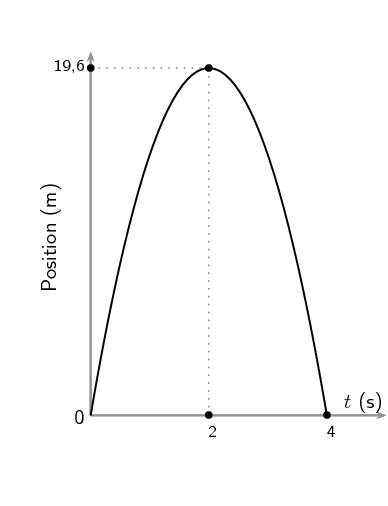### Draw the acceleration versus time graph

To draw the acceleration vs time graph, we need to know what the acceleration is. The velocity versus time graph is a straight line which means that the acceleration is constant. The gradient of the line will give the acceleration.

The line has a negative slope (goes down towards the left) which means that the acceleration has a negative value.

Calculate the gradient of the line:

Therefore the acceleration = $$\text{9,8}$$ $$\text{m·s ^{-2}}$$ downwards.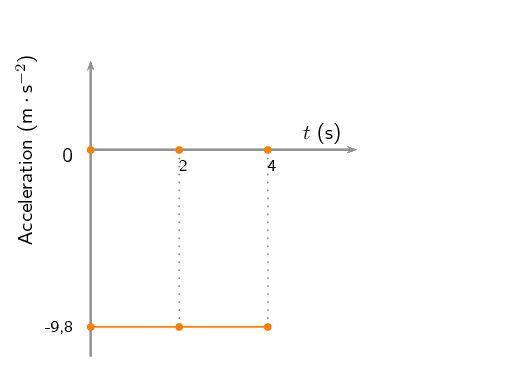## Worked example 7: Interpreting velocity graphs

Describe the motion corresponding to the following graph and plot the position vs time and acceleration vs time assuming the initial position is $$\text{0}$$: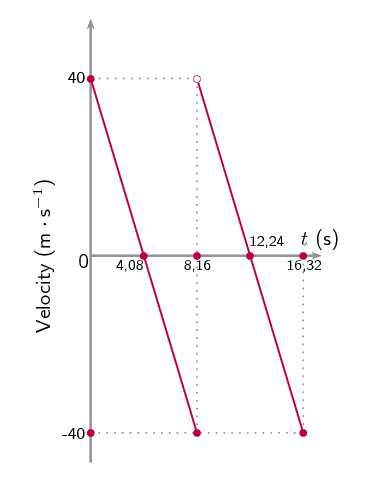### Determine what is required

The velocity vs time graph corresponding to the motion of an object is given, using the graph we need to describe the actual motion. Notice that the graph has discontinuities, this naturally breaks the graph into phases. We should process each phase separately and then try to infer (figure out) what probably happened to change from one phase to the next.

The first thing that you normally do in a vertical projectile motion problem is choose a positive direction. In this case you can't do that because it has already been decided. We need to use the information given to figure out which direction was chosen as positive.

### Determine the direction chosen as positive

We are given a velocity vs time graph. We know that the slope of the velocity time graph is the acceleration and we know that in these problems we are only dealing with gravitational acceleration. We can use what we know about gravitational acceleration and the slope of the graph to determine which direction was chosen as positive.

Gravitational acceleration is always directed towards the centre of the Earth. If we choose:

• upwards as the positive direction then the slope of the velocity vs time graph will be negative
• downwards as the positive direction then the slope of the velocity vs time graph will be positive

In this problem the slope of the velocity vs time graph is negative and so we know that upwards was chosen as the positive direction.

### First phase

In the plot below we highlight only the first phase of interest.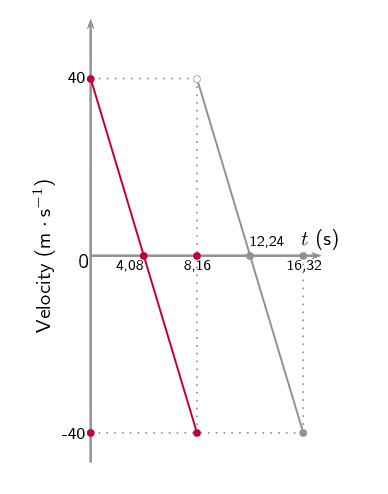The initial velocity is positive and then decreases linearly, passes through zero and reaches the same magnitude in the negative direction. The positive starting value means the object was going upwards, the linear decreases means a constant acceleration in the negative direction, passing through zero means that the object reaches a maximum height before beginning to fall in the negative direction. The fact that the magnitude of the initial and final velocities is the same means that there is time symmetry.

This is just the plot of an object being thrown/shot/projected upwards, peaking and falling back to the same level as it was thrown from.

### Second phase

In the plot below we highlight only the second phase.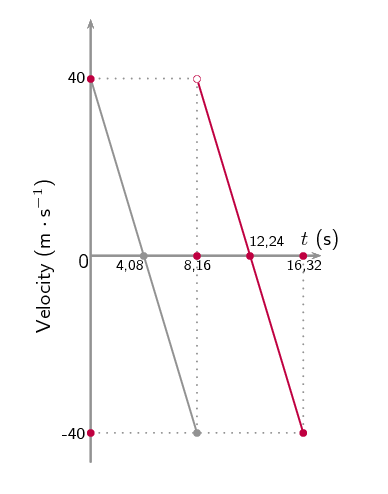Just as in the first phase, the initial velocity is positive and then decreases linearly, passes through zero and reaches the same magnitude in the negative direction. The positive starting value means the object was going upwards, the linear decreases means a constant acceleration in the negative direction, passing through zero means that the object reaches a maximum height before beginning to fall in the negative direction. The fact that the magnitude of the initial and final velocities is the same means that there is time symmetry.

This is just the plot of an object being thrown upwards, peaking and falling back to the same level as it was thrown from.

### Combining phases

We know that the two phases look the same, the object starts off moving upwards, peaks, falls down to the initial position and then repeats the process. This could be a description of a ball bouncing as an example.

### Position vs time

To draw an accurate position graph we know that we are dealing with the Case 1 situation from earlier. We need to determine the height that the object rises to and then we can plot the position vs time. We know the timing information from the velocity vs time plot.

We can verify that the acceleration is in fact from gravity by using $$\vec{v}_f=\vec{v}_i+\vec{g}t$$:

\begin{align*} \vec{v}_f & =\vec{v}_i+\vec{g}t \\ (-\text{40}) & = (\text{40}) +\vec{g}(\text{8,16}) \\ (-\text{80}) & = \vec{g}(\text{8,16}) \\ \vec{g} & = \frac{-\text{80}}{\text{8,16}} \\ \vec{g} & = -\text{9,8}\text{ m·s $^{-2}$} \end{align*}

We can work out the height using the equation for displacement:

\begin{align*} \vec{v}_f^2 & =\vec{v}_i^2+2\vec{g}\Delta \vec{x} \\ 0 & =(\text{40})^2+2(-\text{9,8})\Delta \vec{x} \\ \Delta \vec{x} & =\frac{-(\text{40})^2}{(-\text{19,6})} \\ \Delta \vec{x} & = \text{81,63}\text{ m} \end{align*}

So we can now draw the displacement vs time plot: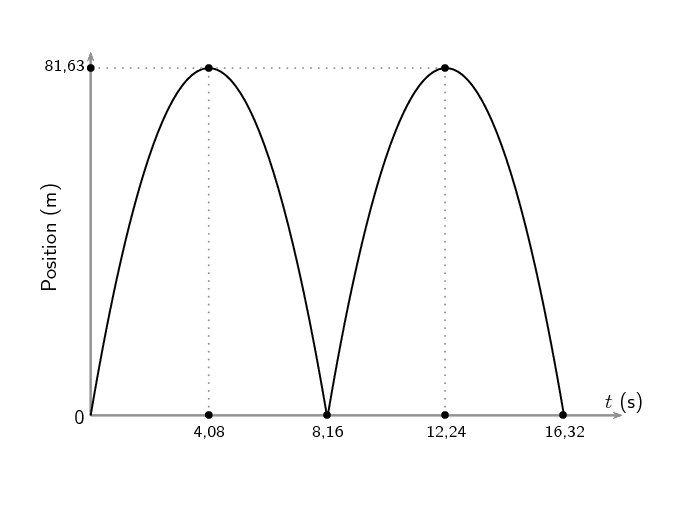### Acceleration vs time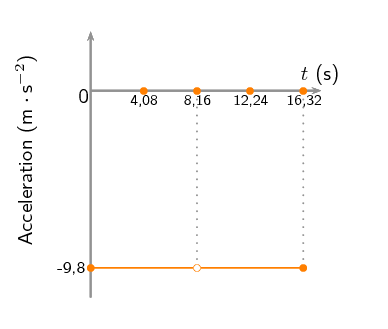The acceleration is due to gravity except at one point, when the object bounces. At that point the acceleration must be some other, very large, value in the positive direction but we don't have enough information to determine what the value must be. We explicitly note that at that point the acceleration is NOT -$$\text{9,8}$$ $$\text{m·s ^{-2}}$$.

## Worked example 8: Hot air balloon [NSC 2011 Paper 1]

A hot-air balloon is moving vertically upwards at a constant speed. A camera is accidentally dropped from the balloon at a height of $$\text{92,4}$$ $$\text{m}$$ as shown in the diagram below. The camera strikes the ground after $$\text{6}$$ $$\text{s}$$. Ignore the effects of friction.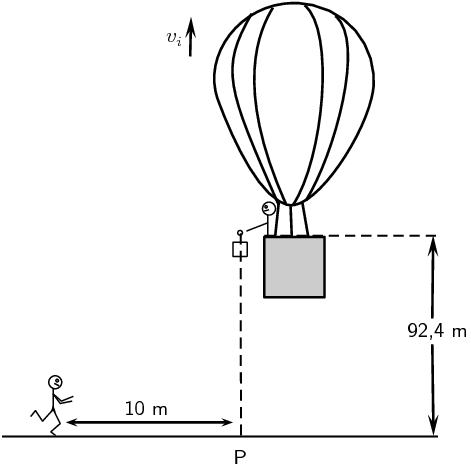1. At the instant the camera is dropped, it moves upwards. Give a reason for this observation.

(1 mark)

2. Calculate the speed $$v_i$$ at which the balloon is rising when the camera is dropped.

(4 marks)

3. Draw a sketch graph of velocity versus time for the entire motion of the camera.

Indicate the following on the graph:

• Initial velocity
• Time at which it reaches the ground

(4 marks)

4. If a jogger, $$\text{10}$$ $$\text{m}$$ away from point P as shown in the above diagram and running at a constant speed of $$\text{2}$$ $$\text{m·s^{-1}}$$, sees the camera at the same instant it starts falling from the balloon, will he be able to catch the camera before it strikes the ground?

Use a calculation to show how you arrived at the answer.

(5 marks)

[TOTAL: 14 marks]

Question 1

The initial velocity / speed of the camera is the same (as that of the balloon).

(1 marks)

Question 2

Taking downwards as the positive direction:

\begin{align*} \Delta y &= v_i \Delta t + \frac{1}{2} a \Delta t^2 \\ \therefore \text{92,4} &= v_i (\text{6}) + \frac{1}{2} (\text{9,8}) (\text{6})^2 \\ \therefore v_i &= -\text{14 m}\cdot\text{s}^{-1} \\ \therefore v_i &= \text{14 m}\cdot\text{s}^{-1} \end{align*}

Taking downwards as the negative direction:

\begin{align*} \Delta y &= v_i \Delta t + \frac{1}{2} a \Delta t^2 \\ \therefore -\text{92,4} &= v_i (\text{6}) + \frac{1}{2} (-\text{9,8}) (\text{6})^2 \\ \therefore v_i &= \text{14 m}\cdot\text{s}^{-1} \end{align*}

(4 marks)

Question 3

Taking downwards as the positive direction: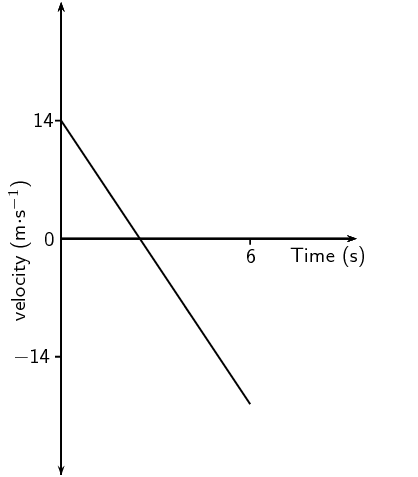Criteria for graph:

• Correct shape as shown. (Straight line with gradient.)
• Graph starts at $$v = \text{14 m}\cdot\text{s}^{-1}\ /\ v_i$$ at $$t = \text{0 s}.$$
• Graph extends below $$t$$ axis until $$t = \text{6 s}.$$
• Section of graph below $$t$$ axis is longer than section above $$t$$ axis.

Taking downwards as the negative direction: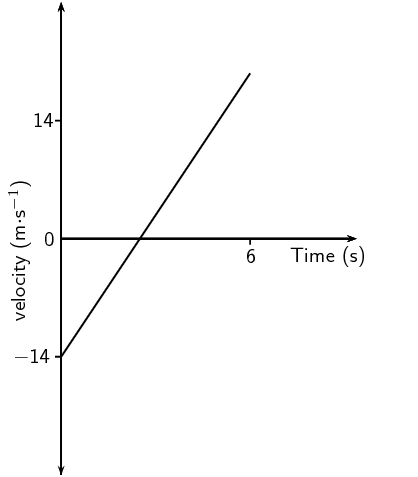Criteria for graph:

• Correct shape as shown. (Straight line with gradient.)
• Graph starts at $$v = -\text{14 m}\cdot\text{s}^{-1}\ /\ v_i$$ at $$t = \text{0 s}.$$
• Graph extends above $$t$$ axis until $$t = \text{6 s}.$$
• Section of graph above $$t$$ axis is longer than section below $$t$$ axis.

(4 marks)

Question 4

Option 1:

\begin{align*} \Delta x &= v \Delta t \\ \therefore \text{10} &= (\text{2}) \Delta t \\ \therefore \Delta t &= \text{5 s} \end{align*}

Yes, he will catch the camera since the time is less than $$\text{6}$$ $$\text{s}$$.

Option 2:

\begin{align*} \Delta x & = v \Delta t \\ \therefore & = (\text{2})(\text{6}) \\ \therefore & = \text{12 m} \end{align*}

Yes, he will catch the camera since the distance covered is greater than $$\text{10}$$ $$\text{m}$$.

Option 3:

\begin{align*} \Delta x & = v_i \Delta t + \frac{1}{2} a \Delta t^2 \\ \therefore \text{10} & = (\text{2})\Delta t + \frac{1}{2}(\text{0})\Delta t^2 \\ \therefore \Delta t & = \text{5 t} \end{align*}

Yes, he will catch the camera since the time is less than $$\text{6}$$ $$\text{s}$$.

Option 4:

\begin{align*} \Delta x & = \left( \frac{v_i+v_f}{2} \right ) \Delta t \\ \therefore \text{10} & = \left( \frac{\text{2}+\text{2}}{2} \right ) \Delta t \\ \therefore \Delta t & = \text{5 t} \end{align*}

Yes, he will catch the camera since the time is less than $$\text{6}$$ $$\text{s}$$.

Option 5:

\begin{align*} \Delta x & = \left( \frac{v_i+v_f}{2} \right ) \Delta t \\ & = \left( \frac{\text{2}+\text{2}}{2} \right )(\text{6}) \\ \therefore \Delta x & = \text{12 m} \end{align*}

Yes, he will catch the camera since the distance covered is greater than $$\text{10}$$ $$\text{m}$$.

(5 marks)

[TOTAL: 14 marks]

## Graphs of vertical projectile motion

Textbook Exercise 3.2

Amanda throws a tennis ball from a height of $$\text{1,5}$$ $$\text{m}$$ straight up into the air and then lets it fall to the ground. Draw graphs of $$x$$ vs $$t$$; $$v$$ vs $$t$$ and $$a$$ vs $$t$$ for the motion of the ball. The initial velocity of the tennis ball is $$2 \text{m}·{\text{s}}^{-1}$$. Choose upwards as positive.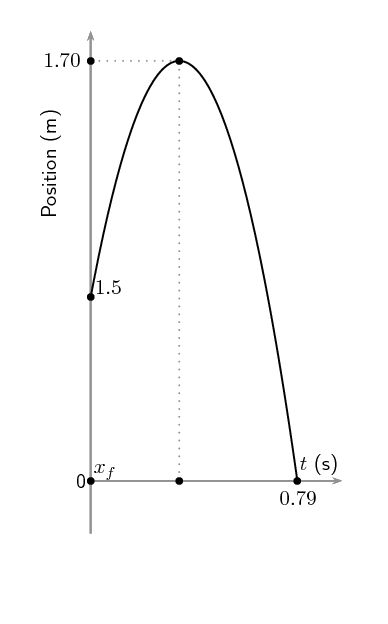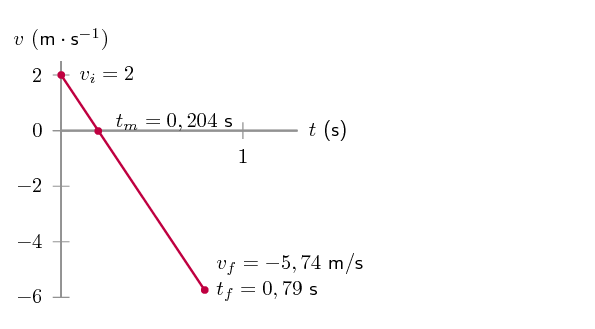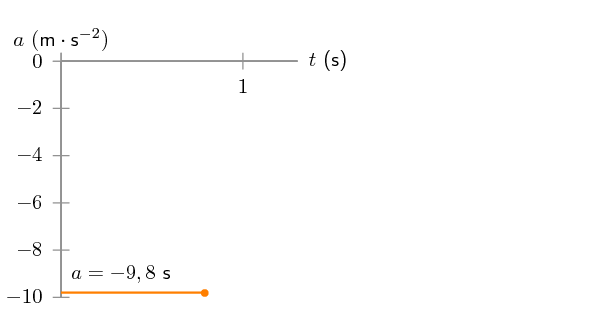A bullet is shot straight upwards from a gun. The following graph is drawn. Downwards was chosen as positive

1. Describe the motion of the bullet.

2. Draw a displacement - time graph.

3. Draw an acceleration - time graph.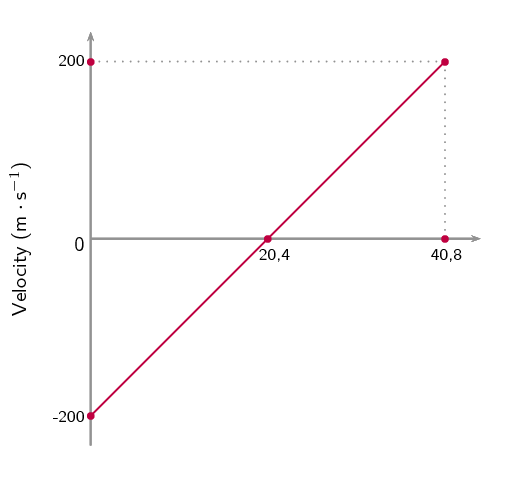1. The bullet moves at a decreasing velocity upwards for $$\text{20,4}$$ $$\text{s}$$. It then stops and drops back down for another $$\text{20,4}$$ $$\text{s}$$ until it reaches a speed of $$\text{200}$$ $$\text{m·s^{-1}}$$ which is the same speed with which it started.

2.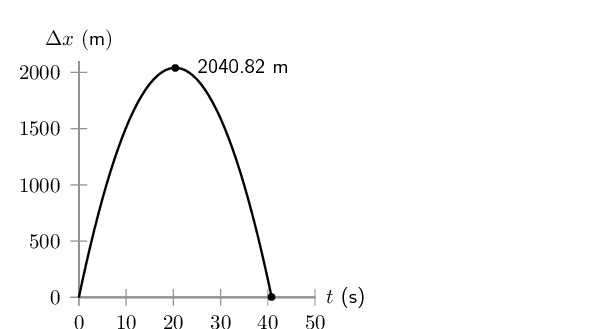3.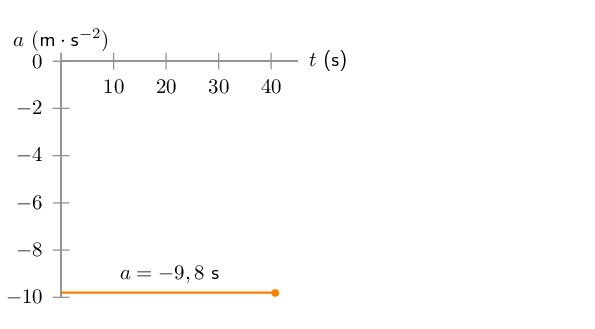Describe the motion resulting from the velocity vs time graph shown below, assuming an initial height of $$\text{0}$$ $$\text{m}$$, and draw a displacement vs time graph and an acceleration vs time graph: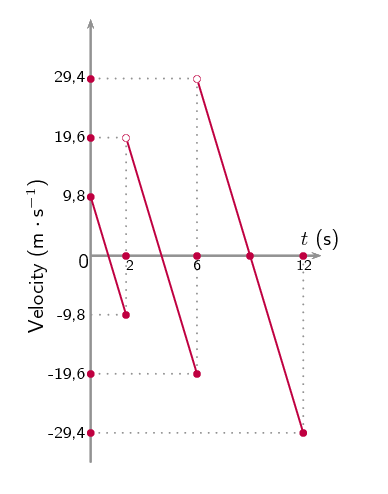The object starts with an initial height of $$\text{0}$$ $$\text{m}$$, moves upwards for $$\text{1}$$ $$\text{s}$$, then slows and falls back to its starting position where it bounces upwards at a higher velocity, and travels upwards (higher than before) for $$\text{2}$$ $$\text{s}$$, then stops and falls back to its starting position where it bounces upwards again at an even higher velocity, travelling over $$\text{3}$$ $$\text{s}$$ to an even higher position, before finally falling back to the ground and stopping.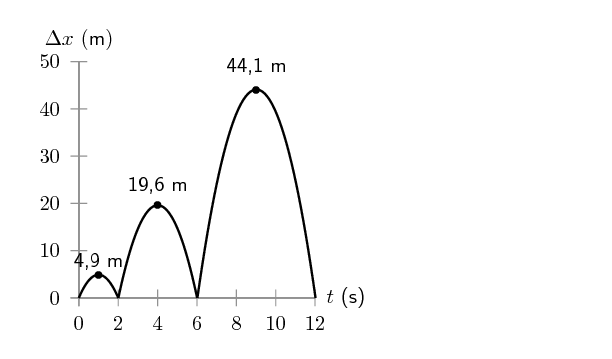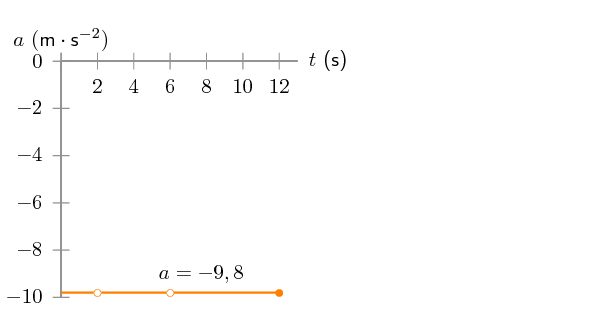What is the initial height? Draw a velocity vs time graph and an acceleration vs time graph that would result in this displacement vs time graph: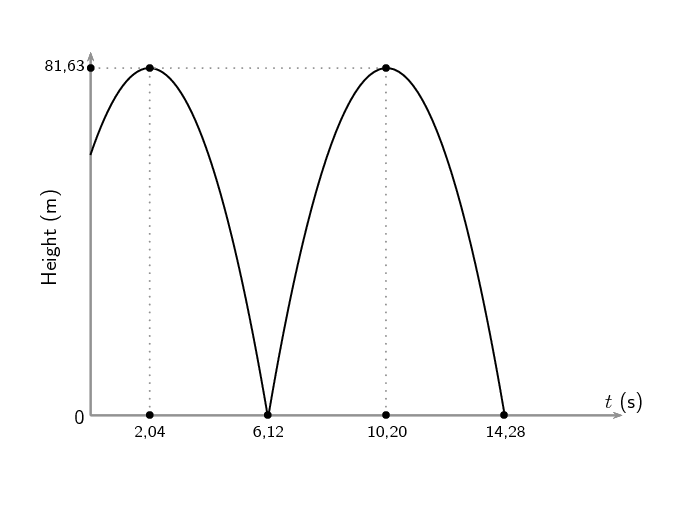Calculate starting height, we can use time symmetry. The initial height is at time zero and the max is at time $$\text{2,04}$$ s. The ball will fall back to the same height as the initial height in $$\text{2,04}$$ s, therefore:

\begin{align*} s &= \frac{1}{2}\vec{g}t^2 \\ &= \frac{1}{2}(\text{9,8})(\text{2,04})^2 \\ &= \text{20,39} \text{ downwards} \end{align*}

Therefore initial height is 81.63-20,39 = 61,24 m.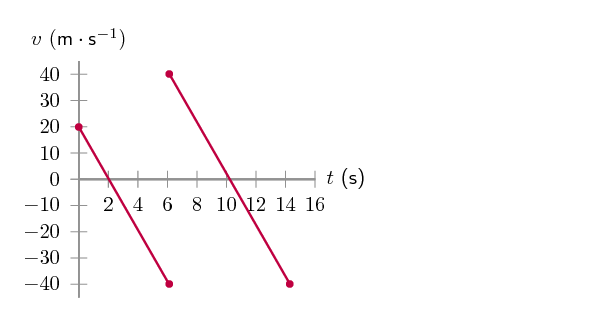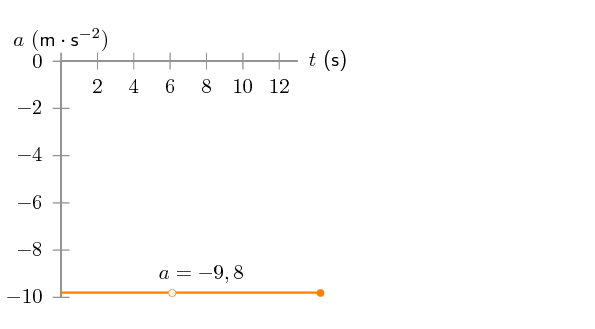A ball that always bounces off the floor with the same magnitude of the velocity as it hit the floor is dropped from $$\text{3}$$ $$\text{m}$$ above a stack of 4 crates. Each crate is $$\text{30}$$ $$\text{cm}$$ high. After the ball bounces off a crate it is quickly removed so that the next time the ball bounces it bounces off the next crate in the pile. This is repeated until all the crates have been removed. Draw the following graphs for the situation:

• Displacement vs time
• Velocity vs time
• Acceleration vs time

The time in each case for the ball to fall from maximum to the crate can be calculated from the distance: \begin{align*} s&=ut+\frac{1}{2}gt^2 \\ &(u=0) \\ s&=\frac{1}{2}gt^2 \\ \frac{2s}{g}&=t^2 \\ t&= \sqrt{\frac{2s}{g}} \end{align*} The ball bounces perfectly which means it always bounces back to the original height. The distances for each bounce are then:

• $$x_1 = \text{3}\text{ m}$$
• $$x_2 = \text{3,3}\text{ m}$$
• $$x_3 =\text{3,6}\text{ m}$$
• $$x_4 =\text{3,9}\text{ m}$$

The times to drop these distances under gravitational acceleration from rest are then:

• $$x_1= \text{3}\text{ m}~ ;~ t_1=\text{0,782460}\text{ s}$$
• $$x_2 = \text{3,3}\text{ m}~ ;~ t_2=\text{0,820651}\text{ s}$$
• $$x_3 =\text{3,6}\text{ m}~ ;~ t_3=\text{0,857142}\text{ s}$$
• $$x_4 =\text{3,9}\text{ m}~ ;~ t_4=\text{0,892142}\text{ s}$$
• $$x_1= \text{3}\text{ m}~ ;~ t_1=\text{0,782460}\text{ s}$$
• $$x_2 = \text{3,3}\text{ m}~ ;~ t_2=\text{0,820651}\text{ s}$$
• $$x_3 =\text{3,6}\text{ m}~ ;~ t_3=\text{0,857142}\text{ s}$$
• $$x_4 =\text{3,9}\text{ m}~ ;~ t_4=\text{0,892142}\text{ s}$$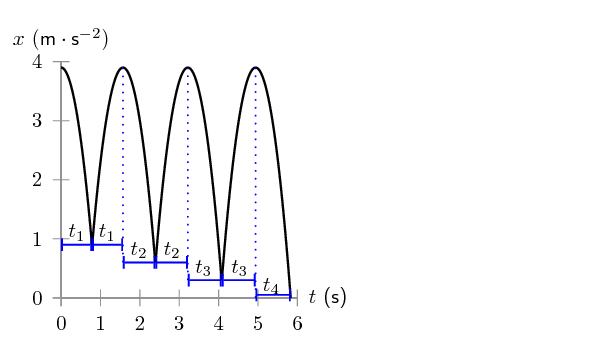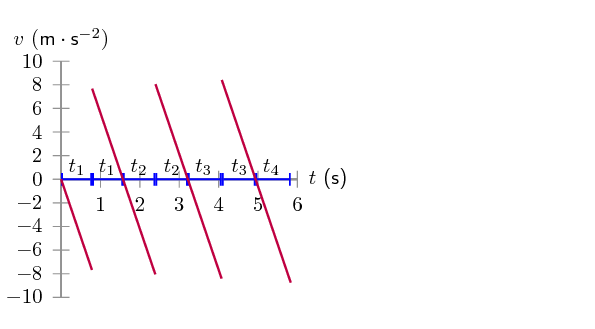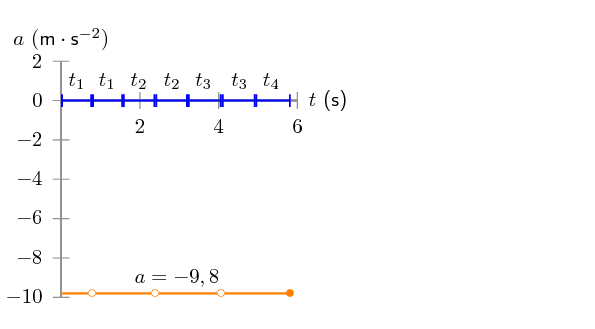A ball is dropped from $$\text{4}$$ $$\text{m}$$ above a cushioned mat. Each time the ball bounces the magnitude of the velocity in the upwards direction is half the magnitude of the velocity with which it hit the floor. The ball is allowed to bounce 2 times, draw the following graphs for the situation and the equation describing each section of the graph:

• Displacement vs time
• Velocity vs time
• Acceleration vs time

The time in each case for the ball to fall from maximum to the mat can be calculated from the distance:

\begin{align*} s&=ut+\frac{1}{2}gt^2 \\ &(u=0) \\ s&=\frac{1}{2}gt^2 \\ \frac{2s}{g}&=t^2 \\ t&= \sqrt{\frac{2s}{g}} \end{align*}

We calculate the velocity with which it hits the mat from:

$v^2 =u^2+2gs$

After the bounce it has an initial velocity upwards of 1/2 the magnitude that it hit with. We use this reduced initial velocity to calculate the new height.

$v^2 =u^2+2gs$
1. First motion:

\begin{align*} v_{1f}^2&=2gs \\ v_{1f}^2&=2(\text{9,8})(4) \\ v_{1f}&=\sqrt{2(\text{9,8})(4)} \\ v_{f1}&= \text{8,854}\ldots\text{ m·s$^{-1}$} \end{align*}

Time to fall from max:

\begin{align*} s_1&=ut+\frac{1}{2}gt_1^2 \\ &(u=0) \\ s_1&=\frac{1}{2}gt_1^2 \\ \frac{2s_2}{g}&=t_1^2 \\ t_1&= \sqrt{\frac{2s_1}{g}} \\ t_1&= \sqrt{\frac{2(4)}{\text{9,8}}} \\ &= \text{0,903}\ldots\text{ s} \end{align*}
2. First bounce, height attained:

\begin{align*} v_{max}^2&=v_{i2}^2+2gs \\ &(v_{max}=0) \\ -(\text{0,5}\times\text{8,854}\ldots)^2&=2(-\text{9,8})s \\ s_2&=\frac{-(\text{0,5}\times\text{8,854}\ldots)^2}{2(-\text{9,8})} \\ s_2&= \text{1}\text{ m} \end{align*}

Time to fall from max:

\begin{align*} s_2&=\frac{1}{2}gt_2^2 \\ &(v_i=0) \\ s_2&=\frac{1}{2}gt_2^2 \\ \frac{2s_2}{g}&=t_2^2 \\ t_2&= \sqrt{\frac{2s_2}{g}} \\ t_2&= \sqrt{\frac{2(1)}{\text{9,8}}} \\ &= \text{0,451}\ldots\text{ s} \end{align*}
3. Second bounce, height attained:

\begin{align*} v_{max}^2&=v_{i3}^2+2gs \\ &(v_{max}=0) \\ -(\text{0,5}\times\text{0,5}\times\text{8,854}\ldots)^2&=2(-\text{9,8})s_3 \\ s_3&=\frac{-(\text{0,5}\times\text{0,5}\times\text{8,854}\ldots)^2}{2(-\text{9,8})} \\ s_3&=\text{0,25}\text{ m} \end{align*}

Time to fall from max:

\begin{align*} s_3&=\frac{1}{2}gt_3^2 \\ &(u=0) \\ s_3&=\frac{1}{2}gt_3^2 \\ \frac{2s_3}{g}&=t_3^2 \\ t_3&= \sqrt{\frac{2s_3}{g}} \\ t_3&= \sqrt{\frac{2(\text{0,25})}{\text{9,8}}} \\ &= \text{0,22587}\ldots \\ &\approx \text{0,226 s} \end{align*}